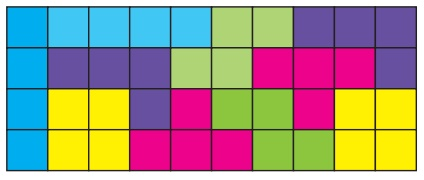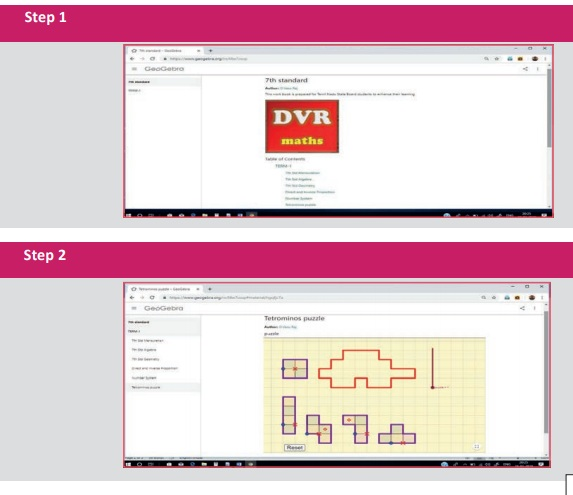Home | | Maths 7th Std | Exercise 6.2 (Route Map)

# Exercise 6.2 (Route Map)

7th Maths : Term 1 Unit 6 : Information Processing : Route Map : Exercise 6.2 : Miscellaneous Practice problems, Challenge Problems, Text Book Back Exercises Questions with Answers, Solution

Exercise 6.2

Miscellaneous Practice problems

1. Make a model of a fish using the given tetromino shapes.2. Complete the given rectangle using the given tetromino shapes.(more possible ways)

3. Shade the figure completely, by using five Tetromino shapes only once.4. Using the given tetrominoes with numbers on it, complete the 4 × 4 magic square ?(more possible ways)

5. Find the shortest route to Vivekanandar Memorial Hall from the Mandapam using the given map.Route 1 : Mandapam → Pullivasal Island → Krusadai Island → Vivekanandar Memorial Hall

6 km + 2 km + 1.5 km = 9.5 km

Route 2 : Mandapam → Krusadai Island → Vivekanandar Memorial Hall

7 km + 1.5 km = 8.5 km

The shortest route to Vivekanandar Memorial Hall from the Mandapam is Mandapam → Krusadai Island → Vivekanandar Memorial Hall

Challenge Problems

6. Fill in 4 × 10 rectangle completely, using all the five tetrominoes twice.(more possible ways)

7. Fill in 8 × 5 rectangle completely, using all the five tetrominoes twice.(more possible ways)

8. Observe the picture and answer the following.(i) Find all the possible routes from A to D.

Route 1 A → G → D

Route 2 A → B → D

Route 3 A → B → C → D

Route4 A → G → F → E → D

(ii) Find the shortest distance between E and C.

The shortest distance between E and C.

E → D → C = 120m + 200m = 320m

(iii) Find all the possible routes between B and F with distance. Mention the shortest route.

Route1   B → A → G → F

250+ 100 + 150 = 500 m

Route2    B → D → E → F

100+ 120 + 300 = 520 m

Route3   B → D → G → F

100 + 200+ 150 = 450 m

Route4   B → C → D → E → F

120 + 200 + 120 + 300 + 740 m

The shortest route is B → D → G → F = 450 m

Exercise 6.25. Mandapam → Krusadai Island → Vivekanandar Memorial Hall

Challenge Problems8 (i)

route 1 A→G→D

route 2 A→B→D

route 3 A→B→C →D

route 4 A→G→F→E→D

(ii) 320 m

(iii) route 1 B→A→G →F

250 100 150 = 600m

route 2 B→D→E →F

100 120 300 = 520m

route 3 B → D → G → F

100 200 150 = 450m

route 4 B→C→D →E→F

120 200 120 300 = 740m

Route 3 is the shortest route.

ICT Corner

Expected Result is shown in this pictureStep – 1 : Open the Browser type the URL Link given below (or) Scan the QR Code. GeoGebra work sheet named “7th std Mensuration” will open. In the right side of the work sheet there are three sliders to change Length, Breadth and Corner angle.

Step - 2 : There are several work sheets for each chapter. Select the worksheet “Tetrominoes Puzzle”. Move the pieces to fit the given shape by dragging the blue points. Red cross can be selected to rotate the pieces. By moving the Red diamond you can flip the pieces. ‘Reset’ button is used to get back to original applet.